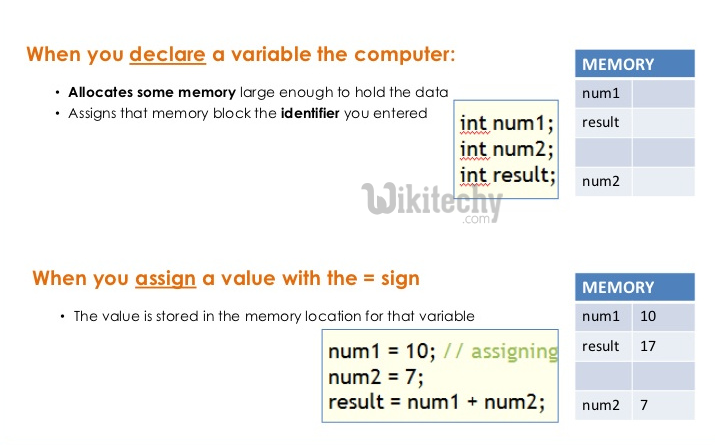# Declaring Variables in Java

## Variables in Java

• A variable is a container that holds values that are used in a Java program.
• Use a variable it needs to be declared. Declaring variables is normally that happens in any program.Learn Java - Java tutorial - Declaring Variables in Java - Java examples - Java programs

## How to Declare a Variable

• Java is a strongly typed programming language.For example, a variable could be declared to use one of the eight primitive data types: byte, short, int, long, float, double, char or boolean.
• We can fill it to a certain level, we can replace what's inside it, and sometimes we can add or take something away from it.
• When we declare a variable to use a data type it's like putting a label on the bucket that says what it can be filled with. Let's say the label for the bucket is "Sand". Once the label is attached, we can only ever add or remove sand from the bucket. Anytime we try and put anything else into it, we will get stopped by the bucket police. In Java, you can think of the compiler as the bucket police. It ensures that programmers declare and use variables properly.
• To declare a variable in Java, all that is needed is the data type followed by the variable name:
• In the above example, a variable called "numberOfDays" has been declared with a data type of int. Notice how the line ends with a semi-colon.
• The semi-colon tells the Java compiler that the declaration is complete.
• Now that it has been declared, numberOfDays can only ever hold values that match the definition of the data type (i.e., for an int data type the value can only be a whole number between -2,147,483,648 to 2,147,483,647).
• Declaring variables for other data types is exactly the same:

## Initializing Variables

• Before a variable can be used it must be given an initial value. This is called initializing the variable. If we try to use a variable without first giving it a value.
• To initialize a variable we use an assignment statement. An assignment statement follows the same pattern as an equation in mathematics (e.g., 2 + 2 = 4). There is a left side of the equation, a right side and an equals sign (i.e., "=") in the middle. To give a variable a value, the left side is the name of the variable and the right side is the value:
• In the above example, numberOfDays has been declared with a data type of int and has been giving an initial value of 7. We can now add ten to the value of numberOfDays because it has been initialized:
• Typically, the initializing of a variable is done at the same time as its declaration:

## Choosing Variable Names

• The name given to a variable is known as an identifier. The way the compiler knows which variables it's dealing with is through the variable's name.
• There are certain rules for identifiers:
• Reserved words cannot be used.
• They cannot start with a digit but digits can be used after the first character (e.g., name1, n2ame are valid).
• They can start with a letter, an underscore (i.e., "_") or a dollar sign (i.e., "\$").
• You cannot use other symbols or spaces (e.g., "%","^","&","#").
• If a variable holds the price of a book, then call it something like "bookPrice". If each variable has a name that makes it clear what it's being used for, it will make finding errors in your programs a lot easier.
• Finally, there are naming conventions in Java that I would encourage you to use. You may have noticed that all the examples I have given follow a certain pattern. When more than one word is used in combination in a variable name it is given a capital letter (e.g., reactionTime, numberOfDays). This is known as mixed case and is the preferred choice for variable identifiers.